# Catch up on the latest AI articles# MTAD-GAT Using Graph-attention For Multivariate Time Series Anomaly Detection

3 main points
✔️ We are building a new framework taking into account the characteristics of multivariate time series data and the purpose of using the results.
✔️ Presenting a solution for cases where relationships are found between variables, rather than lumping univariate data together, will pave the way for a leap forward not only in space and cloud computing but also in many
✔️ The performance of SOTA is even better than SOTA that has been coming out in the last few years.

written by Hang ZhaoYujing WangJuanyong DuanCongrui HuangDefu CaoYunhai TongBixiong XuJing BaiJie TongQi Zhang
(Submitted on 4 Sep 2020)

Subjects: Machine Learning (cs.LG); Machine Learning (stat.ML)code：## first of all

The framework developed is named MTAD-GAT, as the title of the paper suggests. For time series forecasting using deep learning, we introduced a survey paper in a previous AI SCHOLAR article, " Deep Learning Changes Future Forecasting ". I listed elements such as LSTMs as building blocks and mentioned that deep learning may provide outputs that cannot be obtained with classical methods. However, he did not discuss multivariate data in depth there.

LSTM-based encoders and decoders modeling the restoration probability under normal conditions , and stochastic recurrent networks of multivariate time series using stochastic latent variables  have been proposed. However, there is no paper that explicitly captures the correlation between multivariates, and this paper cuts into that.

## technique

MTAD-GAT considers each univariate time series in a multivariate time series as a feature value and tries to explicitly model the correlation between each feature value. On the other hand, it also models the time dependence within each time series.

The core components of the framework are two graph-attention layers: a feature-value-oriented graph-attention layer and a time-oriented graph-attention layer. The feature-value-oriented graph attention layer captures the causal relationships between multiple feature values. Also, the Time-oriented graph/attention layer captures the dependency relationship on the time axis.

Before inputting the data into these layers, the time series data is 1D-convolution processed to extract high-level feature values.

For Graph Attention, see Graph Attention Networks, where Graph Neural Networks are applied to objects that cannot be captured by a Grid-like structure such as MLPs or CNNs, but Graph Attention Networks are applied to objects that cannot be captured in a Grid-like structure such as MLPs or CNNs, but Graph Neural Networks further increase the flexibility of the model by characterizing each node with self-attention. The above two model layers can be optimized simultaneously by combining the objective functions.

The concatenated hidden layer variables then input to the GRU (Gated Recurrent Unit). It captures the sequence patterns of the time series. The output of the GRU is input to the prediction-based model and the recovery model in parallel. Fully connected network is used for the prediction-based model and VAE (Variational Auto Encoder) is used for the restoration model.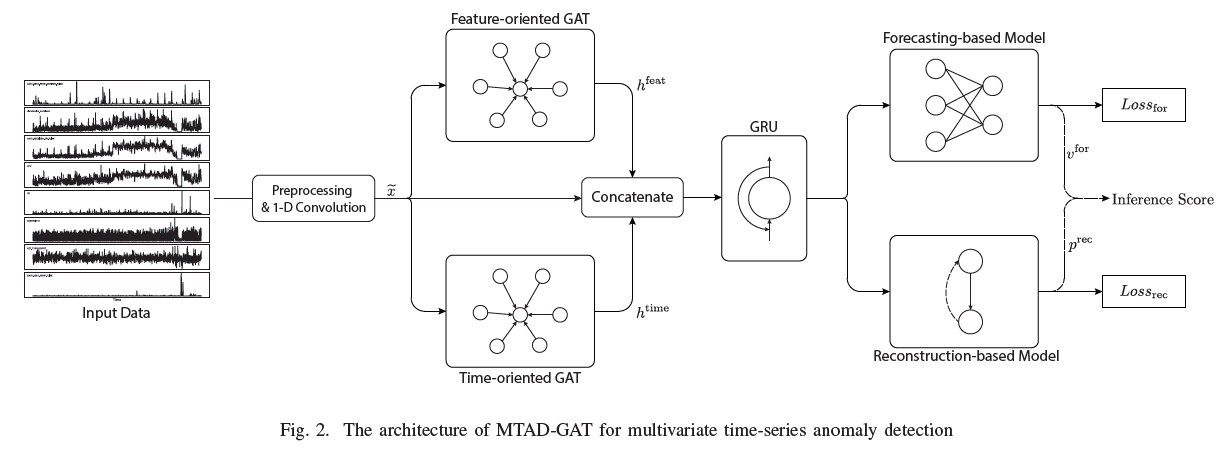### data preprocessing

As preprocessing, we use max-min regularization and SR (Spectral Residual), which is SOTA in univariate anomaly detection.

### graph attention

The graph attention is expressed for each node by the following equation.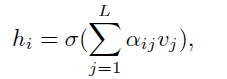σ is the sigmoid activation function; L is the number of nodes in the neighborhood of i. The attention score α is obtained as follows.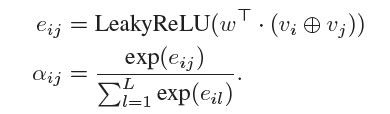1. feature value-oriented graph and attention layer

In the feature value-oriented graph attention layer, a node corresponds to each multivariate variable, and the input is given as a sequential vector of time series data with all timestamps n. The graph is created with k number of variables.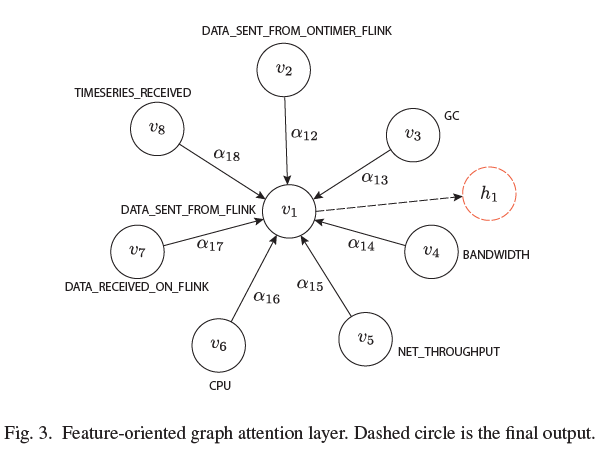2. time-oriented graphs and attention layers

In the time-oriented graph attention layer, time-series data are separated by Sliding windows, there is n number of nodes with n number of timestamps, and vectors with the multivariate number of dimensions are output from each graph.

### joint optimization

The loss function is the prediction model and the loss function of the restoration-based model and the parameters from both models are updated simultaneously.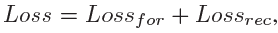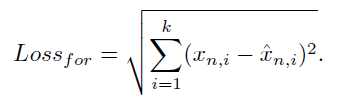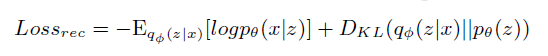The first term in the loss function of the restoration model is the negative log-likelihood and the second term is the Kullback-Leibler divergence.

### model estimation

For each timestamp, there are two inference results. The first is the predicted value, and the second is the restoration probability. For anomaly detection, we balance the two results and use the optimized result. The threshold of the anomaly determination is automatically determined by the POT (Peak Over Threshold) algorithm. The score of the inference is calculated using the hyperparameter γ as follows.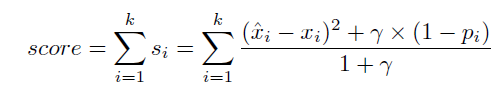## experiment

### Data sets, indicators

Three data sets are used for comparison and evaluation: two are spacecraft data collected by NASA, SMAP (Soil Moisture Active Passive satellite), and MSL (Mars Science Laboratory rover). The other is TSA (Time Series Anomaly detection system) collected by Microsoft's own time series anomaly detection system from a stream processing framework called Flink.

The evaluation metrics are precision, recall, and F1 score.

### Comparison with SOTA

The following eight SOTAs were used for comparison. All of them are multivariate anomaly detection published in 2018-2019. They include prediction-based and restoration-based models.

• Omni-Anomaly .

We propose a probabilistic model for multivariate time-series anomaly detection, in which temporal dependencies are grasped by GRU and then projected onto a probability distribution by VAE. We consider patterns with low recovery probability to be anomalies in comparison with normal patterns.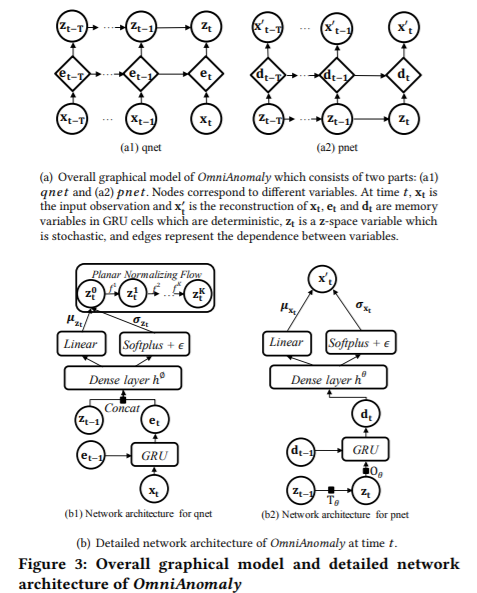• LSTM-NDT .

We propose an unsupervised, non-parameterized threshold determination algorithm for the output of the LSTM.

Feature value extraction, feature value mapping, and anomaly detection are performed through the ensemble of Auto Encoder.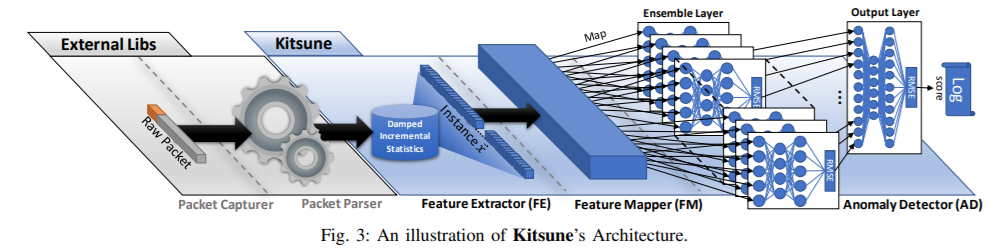• DAGMM .

We do not look at time dependence but focus only on multivariate anomaly detection.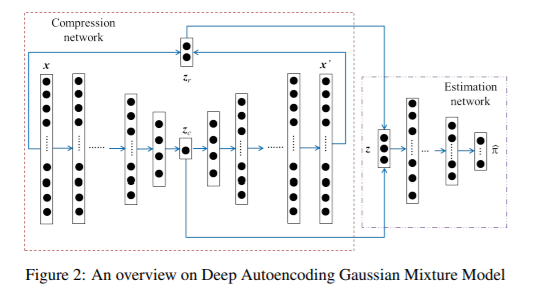By training the Discriminator with GAN, it detects anomalies.